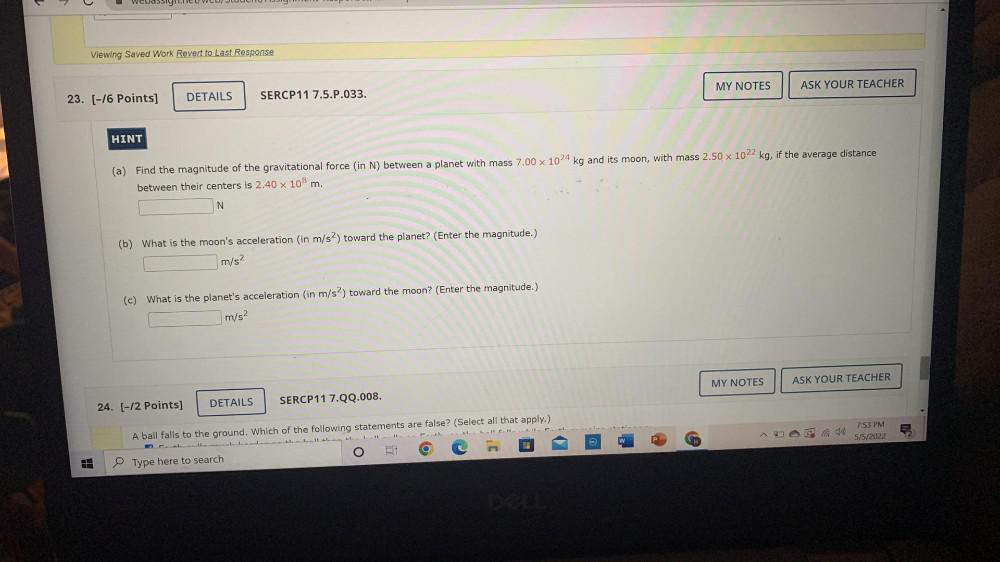Question:

# (a) Find the magnitude of the gravitational force (in N) between a planet with mass 7.00*10²⁴ kg and its moon, with mass 2.50*10²² kg, if the average distance between their centers is 2.40*10⁸ m. (b)(a) Find the magnitude of the gravitational force (in N) between a planet with mass 7.00*10²⁴ kg and its moon, with mass 2.50*10²² kg, if the average distance between their centers is 2.40*10⁸ m. (b) What is the moon's acceleration (in m/s²) toward the planet? (Enter the magnitude.) (c) What is the planet's acceleration in m/s²) toward the moon? (Enter the magnitude.)